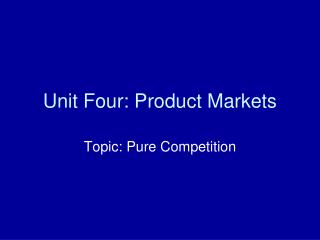# Unit Four: Product Markets - PowerPoint PPT PresentationDownload PresentationUnit Four: Product Markets

Presentation Description
Download Presentation## Unit Four: Product Markets

- - - - - - - - - - - - - - - - - - - - - - - - - - - E N D - - - - - - - - - - - - - - - - - - - - - - - - - - -
##### Presentation Transcript

1. Unit Four: Product Markets Topic: Pure Competition

2. Learning Targets • I will be able to demonstrate how a purely competitive firm is a price-taker, and how it determines output in order to maximize profits or minimize losses.

3. Large # of firms Standardized product “Price-taker” Free entry and exit (no barriers) No advertising Assumptions: All firms have identical costs. Industry is a constant-cost industry (entry and exit of firms will not affect resource prices) Characteristics

4. “Price-Taker” • Def: the firm must sell at industry price. • This implies that: • AR = P (b/c TR = P X Q, so you are adding the price each time you produce an additional unit) • MR = P • Demand curve is perfectly elastic (horizontal) at the given price. • Therefore, MR = D = AR = P! (draw industry and firm graphs)

5. Output Decision • For a firm, it is ideal to continue producing if the benefit (gain) from producing is greater than the cost of producing. • A firm will STOP producing when cost is greater than benefit. • A firm will produce any output where: • TR is greater than or equal to TC (ideally, where it is equal). • MR is greater than or equal to MC (ideally where it is equal).

6. LR Equilibrium • P = MC = minimum ATC (draw) • When economic profit is realized, firms want to enter the industry; this will eliminate the profits and bring the industry back to equilibrium. • When economic loss is realized, firms will want to leave the industry; this will eliminate the losses and bring the industry back to equilibrium. • Normal profit ONLY is realized (minimum income to legitimately stay in business).

7. Efficiency • Productive: least-cost occurs when P = minimum ATC. • Allocative: best-mix occurs when P = MC (if P > MC, resources are being underallocated to the good; if P < MC, resources are being overallocated to the good) • A purely competitive firm is both allocatively and productively efficient in the long-run.

8. SR Profit-Maximization: MR/MC Approach • The optimum quantity to produce (Qe) is where MR = MC. (draw) • Since the firm is a price-taker, price will be set by the industry at MR=D=AR=P. • The area of profit is from where the Qe crosses the ATC up to the price. (draw) • Price must cover AVC in the short-run!

9. Other SR Situations • Loss-minimization: use the MR/MC approach; price must cover AVC in the short run. (draw) • Shut-down: when price does not cover AVC (AVC is above MR=D=AR=P at Qe). (draw)

10. Supply Curve for the Firm • Since P has to cover AVC, the supply curve is the part of the MC curve above the shutdown point (where MC exceeds AVC). (show on graph) • Supply will change the same way it did before (non-price determinants such as resource cost, etc.). • The industry supply curve is the sum of all of the individual firms’ supply curves.

11. YOU MUST REMEMBER: • Price-taker (MR=D=AR=P); elastic/horizontal demand • Qe is at MR=MC. • Profit/loss is at Qe between MR=D=AR=P and ATC. • P must cover AVC to produce. • Long-run equilibrium is achieved when firms enter/exit and MR=MC=minimum ATC. • Full efficiency and normal profit in the long-run.